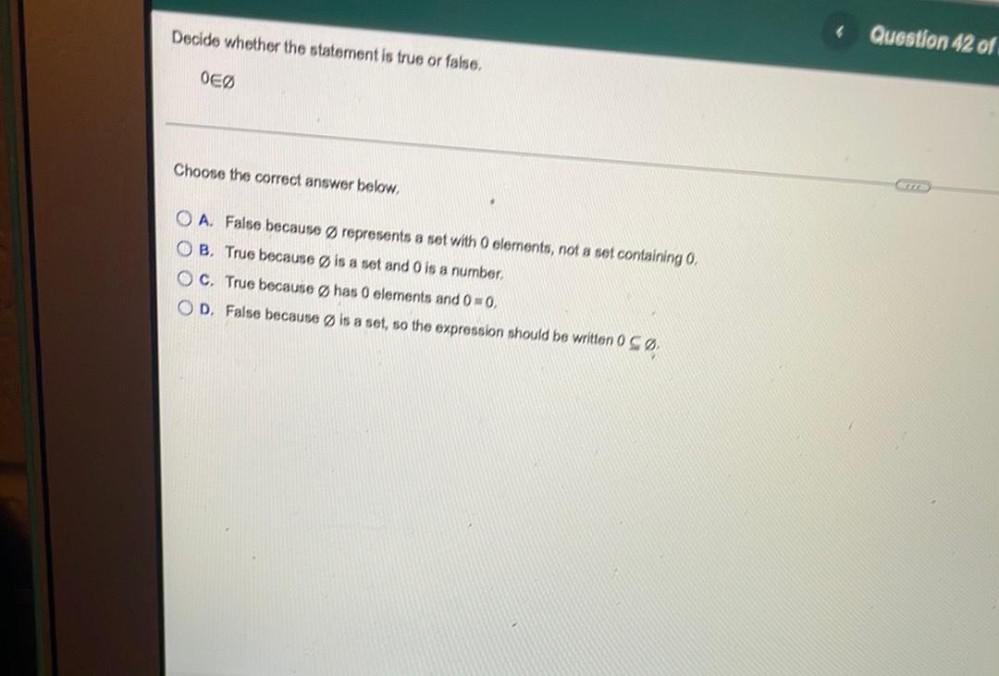Question:

# Decide whether the statement is true or false, Question 42 of OEØ Choose the correct answer below. O A. False because ØrepresentDecide whether the statement is true or false, Question 42 of OEØ Choose the correct answer below. O A. False because Ørepresents a set with O clements, not a set containing , OB True because is a set and Ois a number, OC. True because Øhas 0 elements and 0=0, OD. False because is a set, so the expression should be written 0 SØ